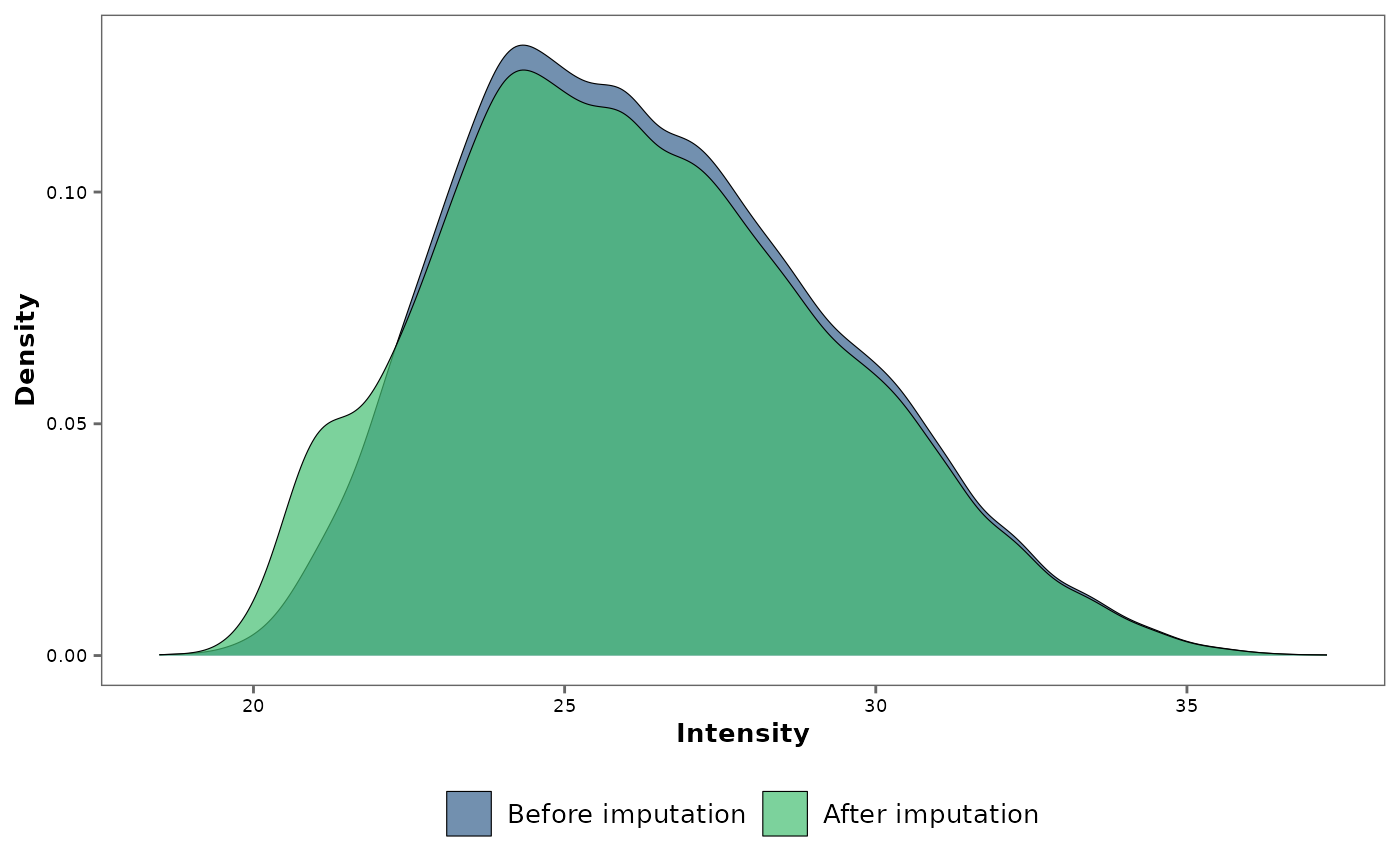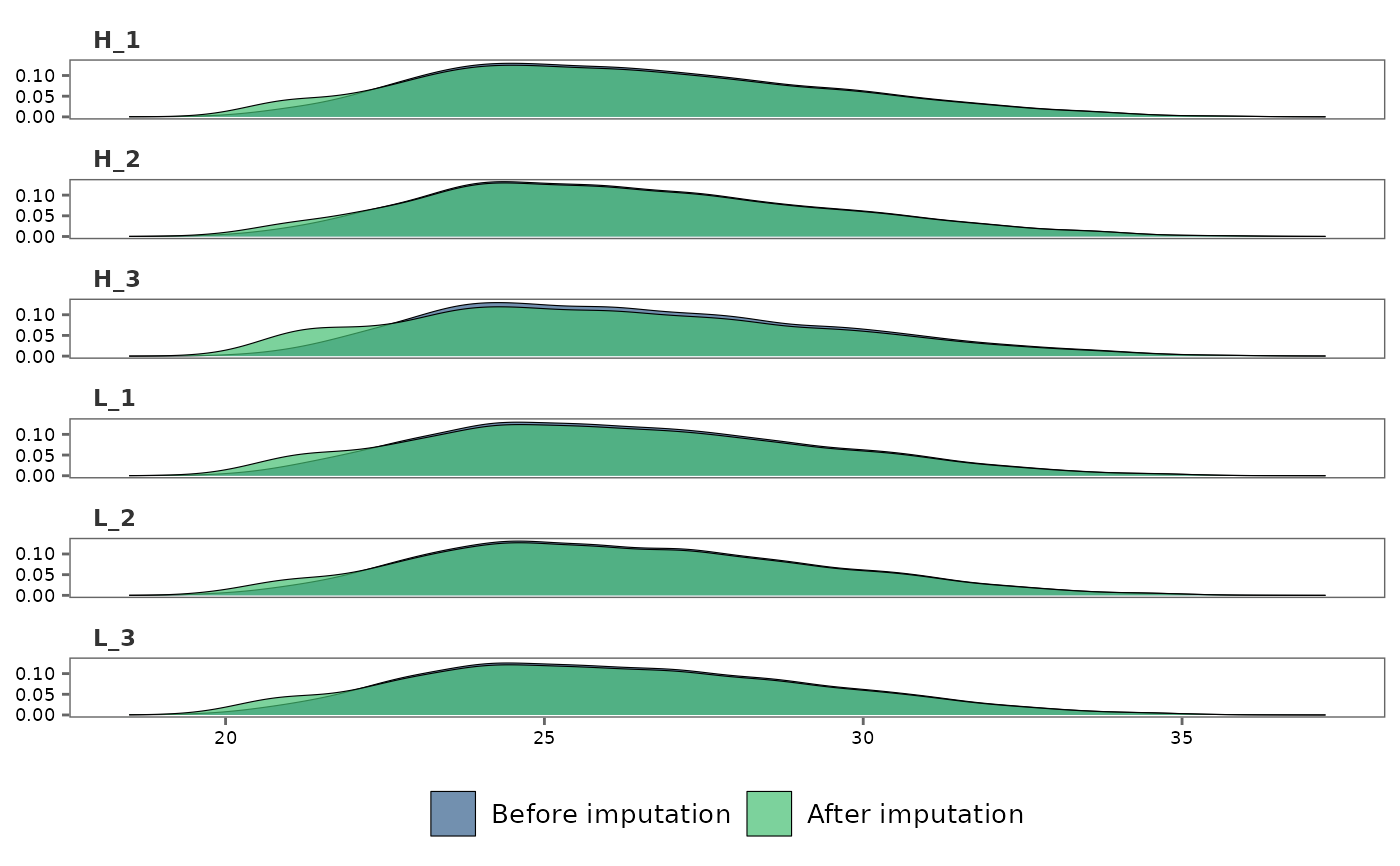This function generates density plots to visualize the impact of missing data imputation on the data.

## Usage

impute_plot(
original,
imputed,
global = TRUE,
text_size = 10,
palette = "viridis",
n_row,
n_col,
save = FALSE,
file_path = NULL,
file_name = "Impute_plot",
file_type = "pdf",
plot_width = 7,
plot_height = 7,
dpi = 80
)

## Arguments

original

A raw_df object (output of create_df) containing missing values or a norm_df object containing normalized protein intensity data.

imputed

An imp_df object obtained from running impute_na on the same data frame provided as original.

global

Logical. If TRUE (default), a global density plot is produced. If FALSE, sample-wise density plots are produced.

text_size

Text size for plot labels, axis labels etc. Default is 10.

palette

Viridis color palette option for plots. Default is "viridis". See viridis for available options.

n_row

Used if global = FALSE to indicate the number of rows to print the plots.

n_col

Used if global = FALSE to indicate the number of columns to print the plots.

save

Logical. If TRUE saves a copy of the plot in the directory provided in file_path.

file_path

A string containing the directory path to save the file.

file_name

File name to save the density plot/s. Default is "Impute_plot."

file_type

File type to save the density plot/s. Default is "pdf".

plot_width

Width of the plot. Default is 7.

plot_height

Height of the plot. Default is 7.

dpi

Plot resolution. Default is 80.

## Value

A ggplot2 plot object.

## Details

• Note, when sample-wise option is selected (global = FALSE), n_col and n_row can be used to specify the number of columns and rows to print the plots.

• If you choose to specify n_row and n_col, make sure that n_row * n_col matches the total number of samples in the data frame.

## Author

Chathurani Ranathunge

## Examples


## Generate a raw_df object with default settings. No technical replicates.
raw_df <- create_df(
prot_groups = "https://raw.githubusercontent.com/caranathunge/promor_example_data/main/pg1.txt",
exp_design = "https://raw.githubusercontent.com/caranathunge/promor_example_data/main/ed1.txt"
)
#> 0 empty row(s) removed.
#> 0 empty column(s) removed.
#> 80 protein(s) (rows) only identified by site removed.
#> 65 reverse protein(s) (rows) removed.
#> 42 protein potential contaminant(s) (rows) removed.
#> 1923 protein(s) identified by 2 or fewer unique peptides removed.
#> Zeros have been replaced with NAs.
#> Data have been log-transformed.

## Impute missing values in the data frame using the default minProb
## method.
imp_df <- impute_na(raw_df)

## Visualize the impact of missing data imputation with a global density
## plot.
impute_plot(original = raw_df, imputed = imp_df)
#> Warning: Removed 1084 rows containing non-finite values (stat_density()).## Make sample-wise density plots
impute_plot(raw_df, imp_df, global = FALSE)
#> Warning: Removed 1084 rows containing non-finite values (stat_density()).## Print plots in user-specified numbers of rows and columns
impute_plot(raw_df, imp_df, global = FALSE, n_col = 2, n_row = 3)
#> Warning: Removed 1084 rows containing non-finite values (stat_density()).